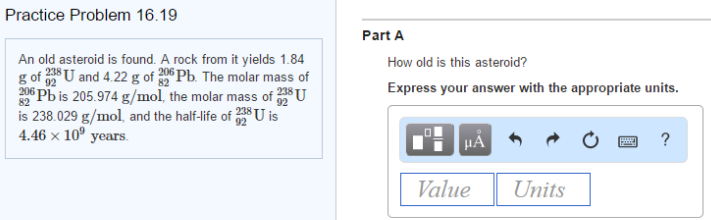# Problem: An old asteroid is found. A rock from it yields 1.84 g of  238U92 and 4.22 g of  206Pb82. The molar mass of 206Pb82 is 205.974 g/mol, the molar mass of  238U92 is 238.029 g/mol, and the half-life of  238U92 is 4.46 x 109 years. How old is this asteroid? Express your answer with the appropriate units.

###### FREE Expert Solution
93% (239 ratings)###### Problem Details

An old asteroid is found. A rock from it yields 1.84 g of  238U92 and 4.22 g of  206Pb82. The molar mass of 206Pb82 is 205.974 g/mol, the molar mass of  238U92 is 238.029 g/mol, and the half-life of  238U92 is 4.46 x 109 years.

How old is this asteroid? Express your answer with the appropriate units.